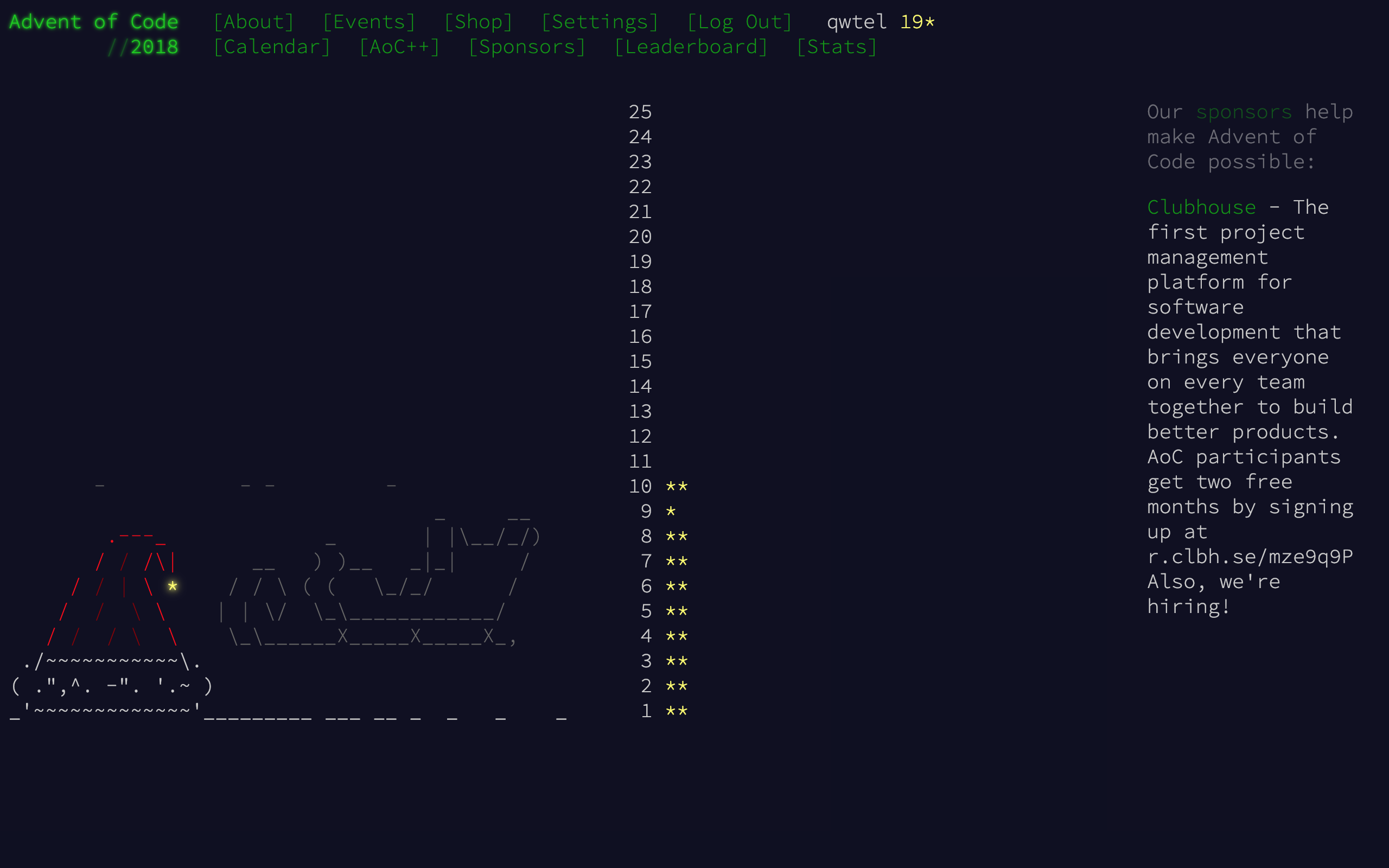# Solving Advent of Code Puzzles with ES GeneratorsJavaScript is not a great langauge for solving programming puzzeles such as Advent of Code, but ECMAScript’s new generator functions may change that.

JavaScript is not a great langauge for solving programming puzzeles such as Advent of Code. It’s lack of a standard library, proper integer type, and general weirdness make it a bad choice for these type of problems.

However, sometimes the tool you know best is best for the task at hand. Since I’ve been mostly using JS recently, I’ve felt more productive using it than any other language, even though I had to write my own functional programming library.

## Iterator Operators

Thanks to generator functions, operators can be implemented quickly and concisely. Not only is it a good learning experience, but it also gives you full knowledge of what’s happening in the background. For example, the ubiquitous map functions is easily implementas as:

function map(f) {
return function* (xs) {
for (const x of xs) yield f(x);
}
}


Note that the inner function is a generator function (indicated by the *) that takes an iterable and returns an iterable. The reason why currying is applied here is to make it usable with a pipe function, so that we can write pipe(x, f, g, h) instead of f(g(h(x))):

const checksum = pipe(
ids,
map(id => frequencies(id)),
map(fqs => [
pipe(fqs.values(), some(x => x === 2)),
pipe(fqs.values(), some(x => x === 3)),
]),
unzip2(),
map(xs => pipe(xs, map(x => x ? 1 : 0), sum())),
reduce((a, b) => a * b, 1),
);


There is a lot going on here — and yes, this is a working solution for Day 2 — but the point is to show that there is a functional programming style that’s made possible by iterators and generators. Each of these operators has an implementation that’s similar to map in both length and complexity.

What’s nice about these iterator-based operators is that intermediate results are never fully realized in memory, e.g. in the example above, at no point is there an array of all frequencies — just a generator with some local state that knows how to produce the next value.

By comparision, an implementation based on Array-methods would look something like this and produce an array of Maps and then another array of tuples, each of which is fully realized in memory, before proceeding to the next step.

ids
.map(id => frequencies(id))
.map(fqs => [
[...fqs.values()].some(x => x === 2),
[...fqs.values()].some(x => x === 3),
]);


## Infinite Sequences

Iterator-based processing is especially handy when dealing with infinite sequences. For example, cycle repeats the provided sequence indefinitly:

const res = pipe(
cycle(input),
scan((a, b) => a + b, 0),
scan((seen, freq) =>
seen.has(freq)
? { reduced: freq }
new Set(),
),
find(({ reduced }) => reduced)
);


What causes the above sequence to come to a stop is find. Once an element is found that meets the condition,the underlying implementation is no longer calling next on the generator up the chain, thereby ending the sequence. Obviously, at no point is an infinite sequence realized in memory.

Just as with map, the implementation of find is rather compact:

function find(p) {
return function (xs) {
for (const x of xs) {
if (p(x)) return x;
}
return null;
}
}


Note that next is implicitly being called as part of the for-of loop.

To show the full awesome might of these oparators, here is an almost complte solution for Day 10:

const RE = /position=<(.*), (.*)> velocity=<(.*), (.*)>/;

const [positions, velocities] = pipe(
lines,
map(line => RE.exec(line).slice(1).map(Number)),
map(([a, b, c, d]) => [[a, b], [c, d]]),
unzip2(),
map(it => [...it]),
);

const [[finalPositions], sec] = pipe(
constantly(velocities),
scan((positions, velocities) => [...pipe(
zip(positions, velocities)
map(([[x, y], [dx, dy]]) => [x + dx, y + dy]),
)],
positions,
),
pairwise(),
zipWith(range(1)),
find(([[prev, curr]]) => {
const prevSize = calcSize(getBounds(prev));
const currSize = calcSize(getBounds(curr));
return currSize > prevSize;
}),
);


Note that sometimes we want to realize a sequence in memory on purpose (achieved by [...x]). Unlike arrays, iterators can only be traversed once, which causes problems when trying to use a result in multiple places. This is one of the major differences between array- and iterator-based processing: With the later, one has to pay attention to the difference between iterators and iterables.

Maybe this is the reason why iterator-based functional programming reamains unpopular in JavaScript. The realiance on a pipe function to make complex pipelines possible is another. Hopefully this will become more practical with the introduction of a pipe operator.

In the meantime, take comfort in the fact that the implementation of pipe is really short as well:

function pipe(x, ...fs) {
let res = x;
for (const f of fs) {
res = f(res);
}
return res;
}


## A Little Iterator Library

I’ve since turned the patterns here into a separate project called lilit (Little Iterator Library), which comes in both an synchronous and asynchronous version. It’s still early but you can try it out like this: